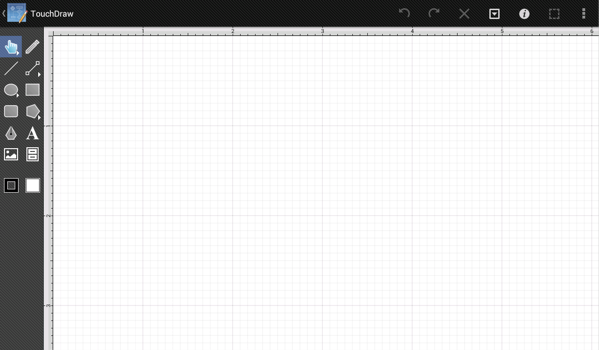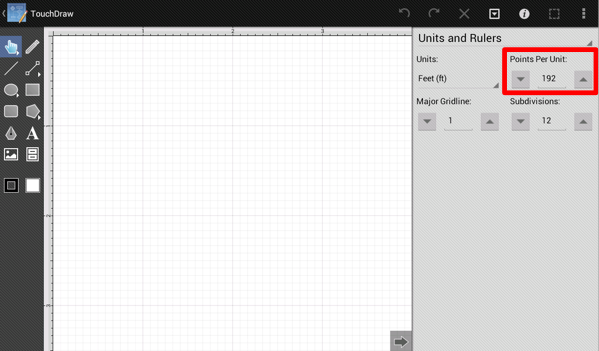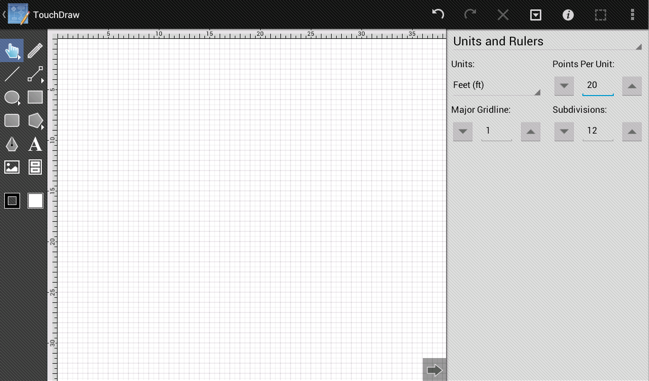# Step 3 - Configure Points Per Unit

After the Unit of Measure has been changed, the Drawing Canvas will have 12 subdivisions for each inch in feet rather than the original 8 subdivisions shown for inches. This is appropriate; however, at 100% zoom only (approximately) 5’ x 3’ are visible on the screen.The scale of the drawing must be adjusted before beginning to draw the floor plan. In TouchDraw for Android this is (currently) done through the Points Per Unit option, which is accessed by opening the Info Menu and then selecting the Units and Rulers option to open the Sidebar as performed in the previous step.The Points per Unit setting is highlighted in the above screenshot. Simple math is necessary to calculate the appropriate value for this setting.

First, the screen resolution used for this Android tutorial is 1024 x 768.

Second, the floor plan being drawn is 30’ x 30’.

Third, taking into consideration that a little bit of a margin will be wanted on the side of the drawing, the drawing view should be approximately 50 feet wide.

Fourth, the appropriate Points per Unit can be calculated by dividing 1024 (the number of horizontal pixels in the screen) by 50 (the width of the drawing in feet).

This calculation supplies a value of 20.48; however, we can simply round to the nearest integer and use 20 for setting the Points Per Unit. If a greater screen resolution will be used such as 1280 x 800, simply divide the first number by 50 to obtain the correct Points Per Unit value, which provides the value of 25.6 or 27 (rounded up). For the sake of simplicity, the traditional screen resolution of 1024 x 768 is used in this tutorial.

Tap on the Down Button next to the Points Per Unit setting in the Units and Rulers Panel to decrease this value by one or tap on the value itself to type in the new Points Per Unit value.A future version of TouchDraw will perform the above calculation automatically by allowing the scale to be selected from a list.

Continue to Next Step